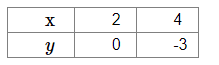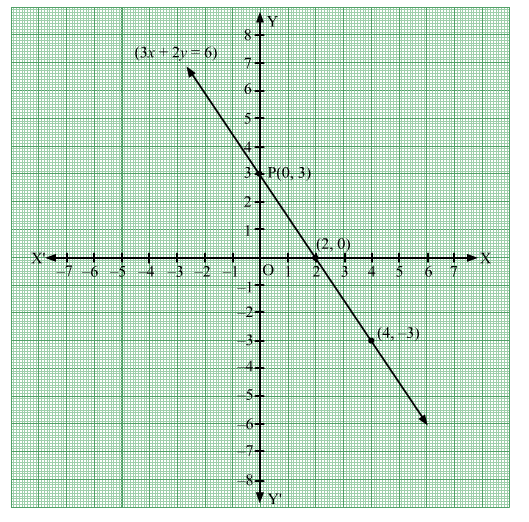# Draw the graph of the equation 3x + 2y = 6. Find the coordinates of the point,

Question:

Draw the graph of the equation 3x + 2y = 6. Find the coordinates of the point, where the graph cuts the y-axis.

Solution:

Given equation: $3 x+2 y=6$. Then,

$2 y=6-3 x \Rightarrow y=\frac{6-3 x}{2}$

When $x=2, y=\frac{6-6}{2}=0$

When $x=4, y=\frac{6-12}{2}=-3$

Thus, we get the following table:Plot the points $(2,0),(4,-3)$ on the graph paper. Join the points and extend the graph in both the directions.Clearly, the graph cuts the $y$-axis at $\mathrm{P}(0,3)$.